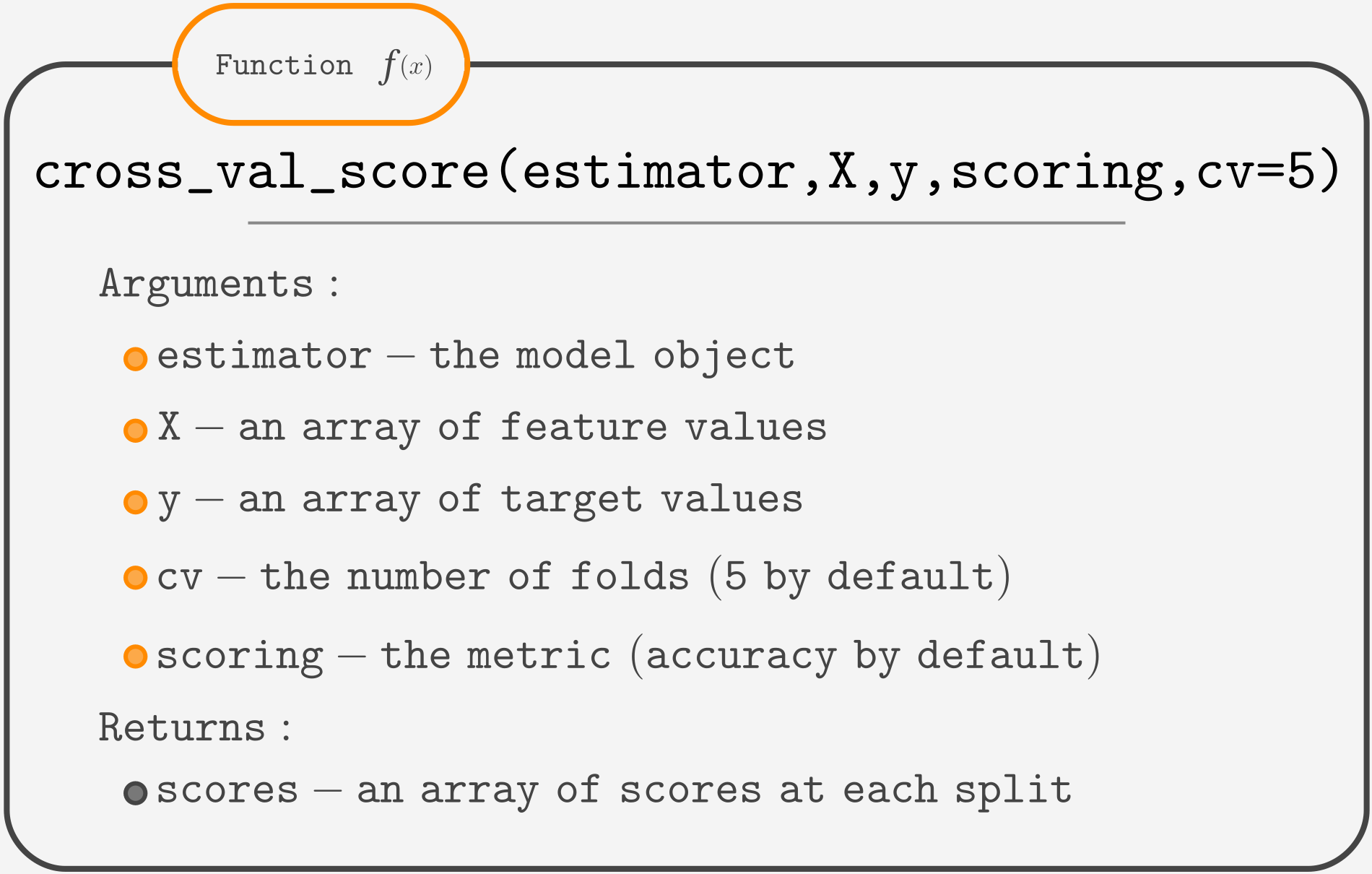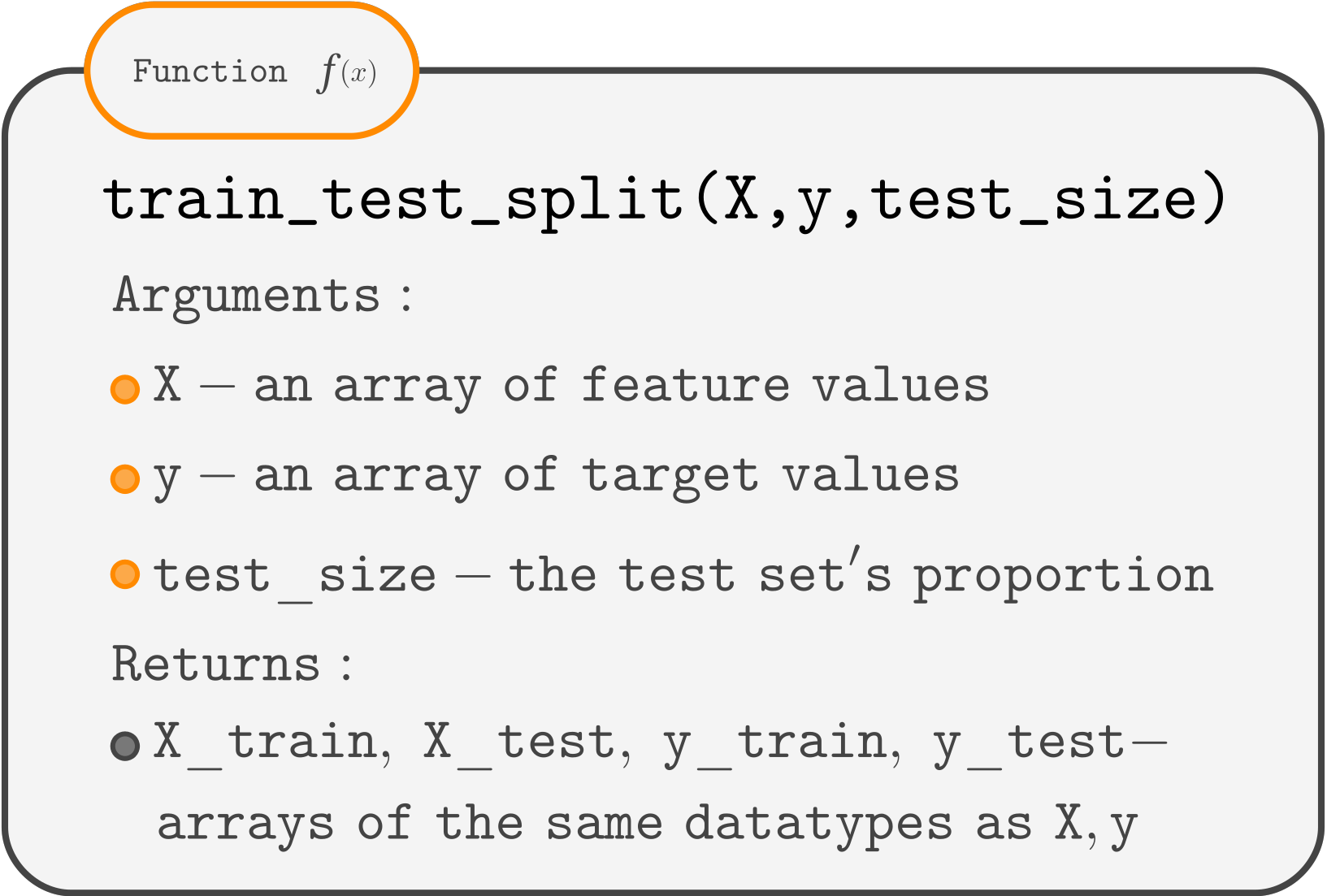Course Content

ML Introduction with scikit-learnCross-Validation Challenge

In this challenge, you will build and evaluate a model using both train-test evaluation and cross-validation.
The data is an already preprocessed Penguins dataset.
Some functions you will use:Build a 4-nearest neighbors classifier and evaluate its performance using the cross-validation score first, then split the data into train-test sets, train the model using the training set, and evaluate it using the test set.

1. Initialize a `KNeighborsClassifier` with 4 neighbors.
2. Calculate the cross-validation scores of this model with the number of folds set to 3.
Note: you can pass an untrained model to a `cross_val_score()` function.
3. Use a suitable function to split `X, y`.
4. Train the model using the training set.
5. Evaluate the model using the test set.

Everything was clear?

Section 4. Chapter 5